• functionvarargout=ode45(ode,tspan,y0,options,varargin)%ODE45Solvenon-stiffdifferentialequations,mediumordermethod.%[TOUT,YOUT]=ODE45(ODEFUN,TSPAN,Y0)withTSPAN=[T0TFINAL]integrates%the...
functionvarargout = ode45(ode,tspan,y0,options,varargin)%ODE45  Solve non-stiff differential equations, medium order method.%   [TOUT,YOUT]=ODE45(ODEFUN,TSPAN,Y0)withTSPAN=[T0TFINAL]integrates%   the system of differential equationsy' = f(t,y) from time T0 to TFINAL%   with initial conditions Y0. ODEFUN is a function handle. For a scalarT%   and a vector Y, ODEFUN(T,Y) must return a column vector corresponding%   to f(t,y). Each row in the solution array YOUT corresponds to a time%   returned in the column vector TOUT.  To obtain solutions at specific%   times T0,T1,...,TFINAL (all increasing or all decreasing), use TSPAN =%   [T0 T1 ... TFINAL].%%   [TOUT,YOUT] = ODE45(ODEFUN,TSPAN,Y0,OPTIONS) solves as above withdefault%   integrationpropertiesreplacedbyvaluesinOPTIONS,anargumentcreated%   withtheODESETfunction.SeeODESETfordetails.Commonlyusedoptions%   arescalarrelativeerrortolerance'RelTol'(1e-3bydefault)andvector%   ofabsoluteerrortolerances'AbsTol'(allcomponents1e-6bydefault).%   If certain components of the solution must be non-negative, use%   ODESET to set the 'NonNegative' property to the indices of these%   components.%%   ODE45 can solve problems M(t,y)*y' = f(t,y) with mass matrix M that is%   nonsingular.UseODESETtosetthe'Mass'propertytoafunctionhandle%   MASSifMASS(T,Y)returnsthevalueofthemassmatrix.Ifthemassmatrix%   is constant, the matrix can be used as the value of the 'Mass' option.If%   themassmatrixdoesnotdependonthestatevariableYandthefunction%   MASS is to be called with one input argument T, set 'MStateDependence'to%   'none'.ODE15SandODE23Tcansolveproblemswithsingularmassmatrices.%%   [TOUT,YOUT,TE,YE,IE]=ODE45(ODEFUN,TSPAN,Y0,OPTIONS)withthe'Events'%   property in OPTIONS set to a function handle EVENTS, solves as above%   while also finding where functions of (T,Y), called event functions,%   are zero. For each function you specify whether the integration is%   to terminate at a zero and whether the direction of the zero crossing%   matters. These are the three column vectors returned by EVENTS:%   [VALUE,ISTERMINAL,DIRECTION]=EVENTS(T,Y).FortheI-theventfunction:%   VALUE(I)isthevalueofthefunction,ISTERMINAL(I)=1iftheintegration%   is to terminate at a zero of this event function and 0 otherwise.%   DIRECTION(I)=0ifallzerosaretobecomputed(thedefault),+1ifonly%   zeroswheretheeventfunctionisincreasing,and-1ifonlyzeroswhere%   the event function is decreasing. Output TE is a column vector of times%   at which events occur. Rows of YE are the corresponding solutions, and%   indices in vector IE specify which event occurred.%%   SOL = ODE45(ODEFUN,[T0 TFINAL],Y0...) returns a structure that can be%   used with DEVAL to evaluate the solution or its first derivative at%   any point between T0 and TFINAL. The steps chosen by ODE45are returned%   in a row vector SOL.x.  For each I, the column SOL.y(:,I) contains%   thesolutionatSOL.x(I).Ifeventsweredetected,SOL.xeisarowvector%   of points at which events occurred. Columns of SOL.ye are thecorresponding%   solutions, and indices in vector SOL.ie specify which event occurred.%%   Example
展开全文• 8.7 常微分方程数值解的若干Matlab函数文件; function [x,y]=euler(f,tspan,y0,n) % 解初值问题 =f(x,y,y(a)=y0 % 使用 n 步的 Euler 法步长 h=(b-a)/h a=tspan(1;b=tspan(2;h=(b-a)/h; x=(a+h:h:b; y(1)=y0+h*feval...
• 在给实验室打工的过程中，遇到了一个需要用到时变参数的微分方程组，解决这种问题利用simulink很好解决，但是项目要求使用m文件进行编程，在以往的学习中，m文件的积分函数一般就是使用ode45，变步长积分器。...
目录写在前面ode45积分器带有时变参数的ode45积分总结写在后面
写在前面
本人大四狗一名，最近在帮实验室肝项目，毕设用的强化学习暂且放下了一段时间，所以没有更新。在给实验室打工的过程中，遇到了一个需要用到时变参数的微分方程组，解决这种问题利用simulink很好解决，但是项目要求使用m文件进行编程，在以往的学习中，m文件的积分函数一般就是使用ode45，变步长积分器。常用的语法是
[t,y] = ode45(@odefun,tspan,y0)
[t,y] = ode45(@odefun,tspan,y0,options)
[t,y,te,ye,ie] = ode45(@odefun,tspan,y0,options)
sol = ode45(___)

这里面有积分时间域，初值以及积分器的设置，我们要做的就是把微分方程组输入到odefun中然后利用ode45进行积分，积分的过程相对是一个黑盒子，在我以往的学习过程中没有把黑盒子拆开了看明白，在解决时变微分方程组时就遇到了问题，我经过搜索相关问题，没有发现网络上给出很好的解答，于是把我研究的一点小东西写下来，给各位同仁分享，希望大家共同进步，另外，如果这边文章对您的工作生活有所用处，还是请您给个点赞关注评论。
ode45积分器
对matlab有所了解的同志应该知道，matlab是一款除了不能生孩子都可以的软件（希望中国能早日有自己的类似软件。），在解决微分方程组的问题时常用simulink搭积木解决，使用m文件相对就会不直观，不容易抓住各种关节，调试起来比较麻烦，但是理解了ode积分器的工作原理之后，也可以很好的进行debug工作。ode45积分器是一个变步长积分器，对很多问题都能很好的解决，我们的抓手其实就是odefun，编辑好微分函数后，具体的积分我们就管不到了，但是在odefun中我们也可以进行一定程度的debug，比较常用的就是把一个语句后面的分号；去掉，来观察我们要看的语句到底有没有运转，运转结果如何，结合我们的猜想进而更改我们的函数。ode45函数具体的使用方法可以在math work中国看他官方给出的help文件，我在此诸摘录一点，
function dydt = odefcn(t,y)
dydt = zeros(2,1);
dydt(1) = y(2);
dydt(2) = 5*y(1);

这就是我们说的odefun，用一个类似状态观测器的形式给出，我们要把这个函数写好，放在matlab可以找到文件夹中。在调用时可以使用
tspan = [0 5];
y0 = [0 0.01];
[t,y] = ode45(@odefcn, tspan, y0);

带有时变参数的ode45积分
时变参数和普通的参数的区别就是他会跟着时间变化，同时ode45是一个变步长积分器，我们怎么知道积分的每一步时间是多少呢?需要变化的参数怎么传递进去呢？怎么把每一步的时间对应到我们需要用到的参数具体值呢？这就需要在odefcn中找到一个抓手，在mathwork中国网站中，给出了时变参数的相关说明，我在此列下来：
ft = linspace(0,5,25);
f = ft.^2 - ft - 3;

gt = linspace(1,6,25);
g = 3*sin(gt-0.25);

function dydt = myode(t,y,ft,f,gt,g)
f = interp1(ft,f,t); % Interpolate the data set (ft,f) at time t
g = interp1(gt,g,t); % Interpolate the data set (gt,g) at time t
dydt = -f.*y + g; % Evaluate ODE at time t
end

tspan = [1 5];
ic = 1;
opts = odeset('RelTol',1e-2,'AbsTol',1e-4);
[t,y] = ode45(@(t,y) myode(t,y,ft,f,gt,g), tspan, ic, opts);

从上面中的例子中我们可以总结出，怎么把参数带进odefcn，用到的语句就是
[t,y] = ode45(@(t,y) myode(t,y,ft,f,gt,g), tspan, ic, opts);

和一般的用法不同，这里我们@的是（t，y）说明函数的时间、初值为t，y，其他的输入是参数，ode45积分器不会使用，这里的参数使用就和其他函数一样了。
！！！！！！！！！！！！！！！！！！！！！
接下来最重要的一点，就是我们在ode45中的抓手，就是（t，y）中的t，光看官网给出的例子，interp1是差分，在ft，f中间差分到t的值，但是我们还是不知道t究竟是一个值还是一个数组，因为我们输入的时间范围，是一个数组。如果interp1差分出来的是积分所有步骤的参数值也说得通，我在最开始也是这么理解的，但是这里的t其实本身就是时变的，并不是一个大数组（和输出的t数组大不同！）在odefcn中循环调用的t就是一个数，代表着本次积分步骤中时间变量。
有了这个理解我们就可以很好地解决时变参数微分方程组积分的问题了，在这里我给出两个解法
1：利用例子中给出的方法，把要使用的参数的一个时间序列输入到odefcn中，在使用中用matlab自身的插值函数来应对变步长不确定的t，这样的方法可能会导致函数精确度下降，因为本身插值就是有误差的，在积分过程中，误差是会累积的，最终形成一个较大误差。
2.如果我们的时变参数不是查表获得，也就是不需要用到插值，也就是有更好的表达方式来获得更加精确的参数值，这种情况下，我们就可以把需要用到的其他参数输入或者global一下变成全局变量，在odefcn中自我求解，这种方式的准确性较高。
我在项目中用到的就是第二种方法，由于项目本身涉密，函数文件不在此展示。
总结
[dy]=odefcn（t,y,a,b）
.........
end

中，t是一个时变数，大小等于积分步骤正在进行的时间大小，y是等式左边的初值，a，b是可以带进来的参数，dy就是y的一阶导数。如果我们需要解决高阶微分方程组问题，可以把ddy成为dy的一阶导，具体思想和状态空间法类似，也和simulink中搭建高阶微分方程组的思想类似。
在得到这个抓手之后，大家就可以根据自身情况来改变函数的内部结构，不把他当作一个黑盒子，拆开来，走进去。
写在后面
由于网上没有这样的详细说明，大佬们都不屑于解释太多，就只能由我这样的菜鸟谈谈想法，有什么理解不到位的地方欢迎和我多交流，共同进步。
水平有限，请有幸看到的您多多指正。在工作之余，祝您早安，午安，晚安。Have a nice day。
（可能的话点个赞再走吧）
P.S.博主还会不定时更新的，喜欢的话就收藏吧。


展开全文• 采用函数句柄传递你定义MATLAB求解器计算的量、例如大规模矩阵或者Jacobian模式的函数。 如果你喜好采用字符串儿传递你的函数matlab求解器将回溯匹配。 在老的matlab版本里，通过传递标志来规定求解器的状态和...
• 计算中立型方程用matlab自带函数sol = ddensd(ddefun,dely,delyp,history,tspan,options)，想请教中立型微分方程的功率谱图，分岔图，lyapunov指数图
• ## Matlab求常微分方程组的数值解

万次阅读 多人点赞 2020-03-08 09:50:50
上篇博客介绍了Matlab求解常微分方程组解析解的方法：博客地址 微分方程组复杂时，无法求出解析解时，...一般形式：[t,y] = ode45(odefun,tspan,y0) 其中 tspan = [t0 tf] 功能介绍：求微分方程组 y′=f(t,y) 从 t0...
上篇博客介绍了Matlab求解常微分方程组解析解的方法：博客地址
微分方程组复杂时，无法求出解析解时，就需要求其数值解，这里来介绍。
以下内容按照Matlab官方文档提供的方程来展开（提议多看官方文档）
介绍一下核心函数ode45()
一般形式：[t,y] = ode45(odefun,tspan,y0)		其中 tspan = [t0 tf]
功能介绍：求微分方程组 y′=f(t,y) 从 t0 到 tf 的积分，初始条件为 y0。解数组 y 中的每一行都与列向量 t 中返回的值相对应。
1. 一阶微分方程求解(简单调用即可)
方程：y’=2*t
代码：
tspan=[1 6]; %定义自变量x的取值空间为1-6
y0=0;%定义因变量的初值，当x=1（x取值空间的第一个数）时，y0=0
[t,y]=ode45(@(t,y) 2*t,tspan,y0); %定义函数y'=2*t,使用ode45求解
plot(t,y,'-o'); %绘制求得的数值曲线

说明：简单的odefun参数就是这个形式，@(x,y) fun	中间是空格，不能用逗号
结果：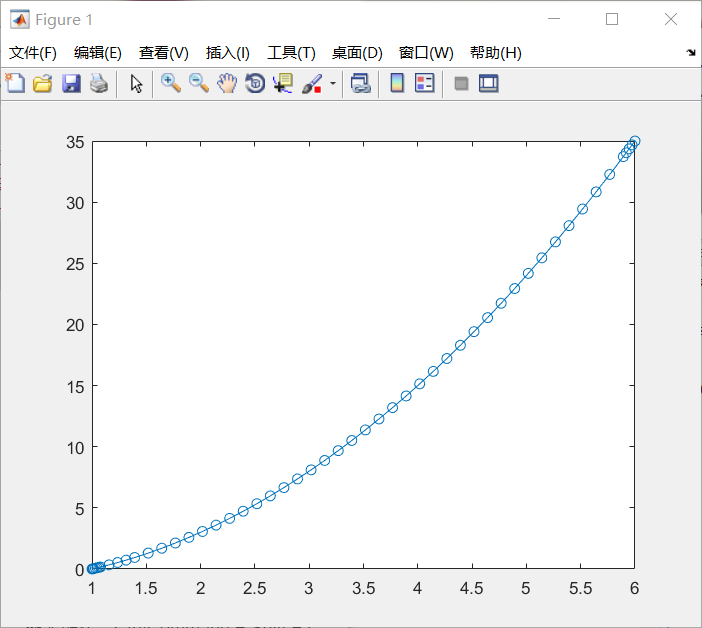2. 二阶微分方程求解(引入函数文件)
方程：范德波尔方程 y1’’-u(1-y1²)*y1’+y1=0;（这里设u=1）
代码：
定义输入的方程，以函数形式定义
function dydt=odefun(t,y)
%二阶方程为y1''-(1-y1²)*y1'+y1=0;
%降阶为两个方程：y1'=y2;
%               y2'=(1-y1²)*y2-y1;
%t虽然没有使用，但必须要作为参数写入
dydt=[y(2);(1-y(1)^2)*y(2)-y(1)];

求解作图
tspan=[0 20]; %定义自变量x的取值空间为0-20
y0=[2;0];%定义因变量的初值，当x=0时，y1=2,y2=y1'=0;
[t,y]=ode45(@odefun,tspan,y0); %使用ode45求解
%%下面为作图过程，不解释
plot(t,y(:,1),'-o',t,y(:,2),'-o')
title('Solution of van der Pol Equation (\mu = 1) with ODE45');
xlabel('Time t');
ylabel('Solution y');
legend('y_1','y_2')

说明：先进行降阶，使y2=y1’，这时候y2’=y1’’，然后把两个方程写成列向量形式就行了
结果：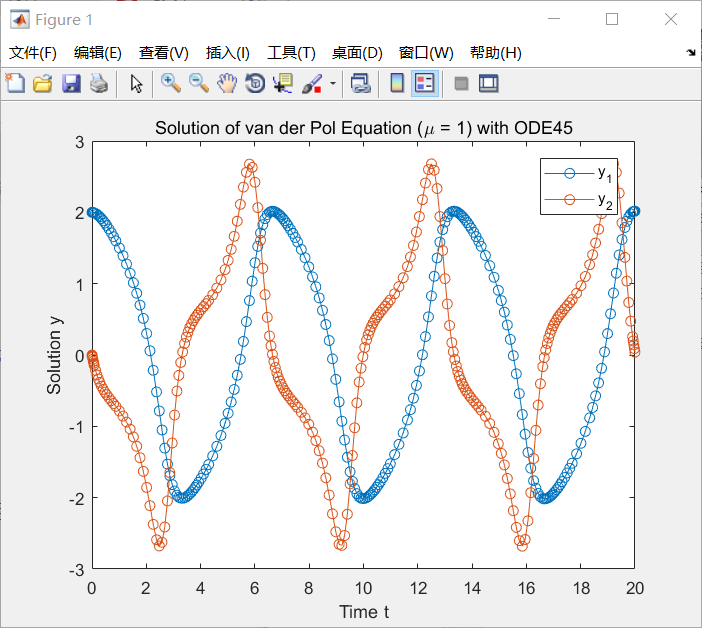3. 求解微分方程组（和2类似）
这里就和求解二阶方程类似的，只不过不需要降阶，仍旧需要一个函数来定义方程组。我们这里不用官方文档的例子，用同学的循坏摆问题来进行演示。
方程：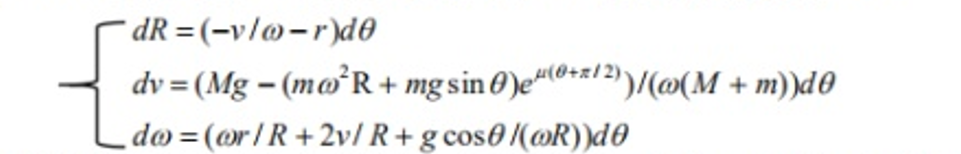给定的初值（w接近0，但实际上不能设置为0）：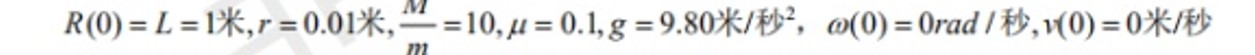代码：
定义输入的方程
function dRvw=func(t,Rvw)
%% 函数功能：为ode45提供微分方程
%输入：t:时间序列，就是θ；Rvw:因变量,Rvw(1)代表R,Rvw(2)代表v,Rvw(3)代表w
%输出：dRvw:因变量的一阶微分,dRvw(1)代表dR,dRvw(2)代表dv,dRvw(3)代表dw
%% 初始化因变量的一阶微分，3×1的向量
dRvw=zeros(3,1);
%% 参数初始化
r=0.01;u=0.1;g=9.8;M=10;m=1;
%% 输入微分方程式
dRvw(1)=-Rvw(2)/Rvw(3)-r;
dRvw(2)=(M*g-(m*Rvw(3)^2*Rvw(1)+m*g*sin(t)) *exp(u*(t+pi/2)))/(Rvw(3)*(M+m));
dRvw(3)=Rvw(3)*r/Rvw(1)+2*Rvw(2)/Rvw(1)+g*cos(t)/(Rvw(3)*Rvw(1));

求解作图
%% 参数初始化 start_Theta是θ的初始值 end_Theta是θ的结束值
%R是半径初值；v是线速度初值；w是角速度初值
start_Theta=0;end_Theta=2*pi;R=1;v=0;w=1e-5;
%% 使用ode45方法计算微分方程组func的数值解
%func是带有方程组的函数
%[start_Theta end_Theta]是自变量范围
%[R;v;w]是方程初值
%T是自变量的数组，Rvw是对应的因变量的数值。Rvw(:,1)=R;Rvw(:,2)=v;Rvw(:,3)=w;
[T,Rvw]=ode45(@func,[start_Theta end_Theta],[R;v;w]);
%% 组合自变量和因变量。TRvw(:,1)=θ;TRvw(:,2)=R;TRvw(:,3)=v;Trvw(:,4);
TRvw=[T,Rvw];
%% 绘制自变量-因变量图像.figure1是R-θ,figure2是v-θ,figure3是w-θ
plot(T,Rvw(:,1),'-o',T,Rvw(:,2),'-o',T,Rvw(:,3),'-o')
title('自变量-因变量图像');
xlabel('Angle θ');
ylabel('Solution');
legend('R','v','w')

说明：注释的应该是比较清楚的，把三个方程写成列向量的形式就行
PS:有些人和我说不能运行，然后我看了他们出错的原因，有点儿哭笑不得。出错的基本上都是运行上面的dRvw=func（t，Rvw）这个函数的。说明一下，这是有参数的函数，不给参数不能直接运行的。下面的求解作图脚本才是需要运行的哈，它调用了函数，才得到的结果。如果大家还发现什么问题，欢迎私戳或评论。
PS+ 有了PS之后，还是很多人问我参数的问题，我在这里直接把文件给大家：cupt.zip
提取码：6k8n。
解压后，有两个文件，大家直接运行cupt.m即可。
结果：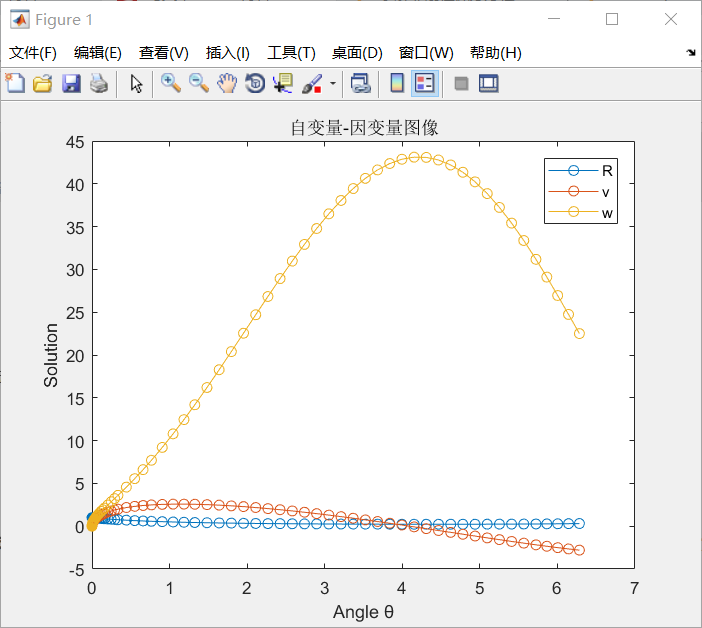4. 更多形式
讲到这里，大部分我们用到的微分方程形式都可以求解了，Matlab还支持带有时变项和额外参数的微分方程求解，这里不再赘述，大家可以自行参阅官方文档。


展开全文经验分享 编程语言 常微分方程
• 首先编写函数文件.m作为ode45的第一个参数odefun function y = fun(t,x0,flag,k) %flag is a [] to mark the args 可略去不写y = fun(t,x0,k) %kequ中参数 %x0初值given %--------------- %some
ode45解微分equation
[t,y] = ode45(odefun,tspan,y0)example
[t,y] = ode45(odefun,tspan,y0,options)example

首先编写函数文件.m作为ode45的第一个参数odefun
function y = fun(t,x0,flag,k)
%flag is a [] to mark the args 可略去不写y = fun(t,x0,k) %kequ中参数
%x0初值given
%---------------
%some global setting
lamda=1;
%---------------
y=[x0(2)*k;
lamda*x0(2)*(1-x0(1)-x0(2))-k*x0(2)];
end

调用：
[t,x]=ode45('fun',tspan,[0,tf],[],params);
%or [t,x]=ode45(@fun,tspan,[0,tf],[],params);

tspan为[0:10] 自变量范围
第三个参数为初值向量
第四个参数空阵组作flag 区别开传入fun的参数和ode45所需要的参数


展开全文• matlab求解时滞微分方程，dde23调用格式： sol = dde23(ddefun,lags,history,tspan); –ddefun函数句柄，求解微分方程y’=f(t,y(t),y(t-τ1),…,y(t-τk)) 必须写成下面形式： dydt =ddefun(t,y,Z); 其中t对应...
• 1.ode23： ...‘F’ 是包括函数文件名字的字符串或函数句柄。函数F(t,y) 必须返回列向量 Tspan = [t0，tN]是常微分方程求解区域；或具体节点. 例:linspace(a,b,step) y0是表示初始条件 例1： function t
• matlab提供了求常微分方程数值解的函数。当难以求得微分方程的解析解时，可以求其数值解. matlab中求微分方程数值解的函数有七个：ode45，ode23，ode113，ode15s，ode23s，ode23t，ode23tb 。 ode45是解决数值解问题...数学建模
• [matlab]数值计算微分方程组当我们使用dsolve无法进行符号求解时，我们使用ode45函数函数原型为[t,y] = ode45(odefun,tspan,y0)example [t,y] = ode45(odefun,tspan,y0,options)example [t,y,te,ye,ie] = ode45...
• [t,y]=solver('odefun',tspan,y0,options)其中solver选择ode45等函数名，odefun为根据待解方程或方程组编写的m文件名，tspan为自变量的区间[t0,tf]，即准备在那个区间上求解，y0表示初始值，options用于设定误差限制...
• 常微分方程数值求解函数3. 常微分方程数值求解函数统一命名格式4. 刚性问题 1. 常微分方程数值求解的一般概念 2. 常微分方程数值求解函数 [t,y]=solver(filename,tspan,y0,option) t：时间向量； y：数值解； ...
• %% 通用函数 [t,y]=main(ddefun,kernelfun,initialfun,lag,tspan,dimensional) %% 其中 ddefun为右端函数，kernelfun为积分核函数，initialfun为初始函数,lag为延迟量， %% tspan为求解区间，dimensional为问题维数 ...
• 函数句柄的创建 1、 直接@ A=@sin(x); A(pi) 2、创建匿名函数 %定义函数f(x)=x^2 f = @(x) x^2; f(2) ODE45使用 [t,y] = ode45(odefun,tspan,y0) t：自变量 y(t):因变量 odefun：函数句柄(自动y...
• 这个函数用来解决微分方程组 先上代码 tspan=[1:0.01:10]； X0=[0;15]； delta=@(x,y) [y(2);-29*y(1)-4*y(2)]； [T,X]=ode45(delta,tspan,X0)； plot(T,X(:,1))； plot(T,X(:,2))； 先声明一
• 我在做演化博弈使用ode45函数求解时出现了如下错误，请问如何解决？ 索引超出矩阵维度。 出错 differential (line 2) dxdt=[x(1)*(1-x(1))*(10-3*x(3)-5*x(2));x(2)*(1-x(2))*(4*x(1)+12.6*x...
• （1）中性类型的时滞微分方程...函数说明：ddefun是微分方程的描述，dely是时滞部分的描述，delyp是时滞导数部分的描述，history初始条件，tspan是求解时t的范围。 （3）例子： 1.所求解方程： 初始状态为：y(t)=cos
• 这是我的函数m文件 2. ` function [ t, y]=RK4(myfun,tspan,y0,h) t=tspan(1):h:tspan(2); y=zeros(length(y0),length(t)); y(:,1)=y0(:); for n=1:(length(t)-1) k1=feval(myfun,t(n),y(:,n)); k2=...开发语言
• ## 四阶龙格库塔法

千次阅读 2019-11-27 19:43:32
四阶龙格-库塔方法的原理 ...Matlab内部函数ode45直接进行常微分方程求解，函数格式为： [X,Y]=ode(odefun,tspan,Y0) Odefun设置为微分方程中需要积分的函数tspan为微分方程积分的范围， Y0为Y的初始值。 结...
• ode45要解的微分方程，有一...这个问题可以用matlab的插值函数，把数值函数变成“解析函数来解决”，这样就能直接套matlab的ode45代码了 [t,y] = ode45(@(t,y) 2*t, tspan, y0); 中有解析函数 2t 它可以用 x1=...
• ode45是matlab中的求解微分方程数值解的函数，使用语法为： [t,y] = ode45(odefun,tspan,y0) [t,y] = ode45(odefun,tspan,y0,options) [t,y,te,ye,ie] = ode45(odefun,tspan,y0,options) sol = ode45(___) odefun...
• 分析：该方程应该在某个t0时间之后成立，初始值必须是定义在t0之前的一个关于t的单值向量函数phi(t)。 我假设t0=0吧，phi(t)=[1;-1]; matlab程序： function ddeex t0 = 0; tfinal = 5; tspan = [t0, tfinal]; sol ...# matlabtspan函数matlab 订阅